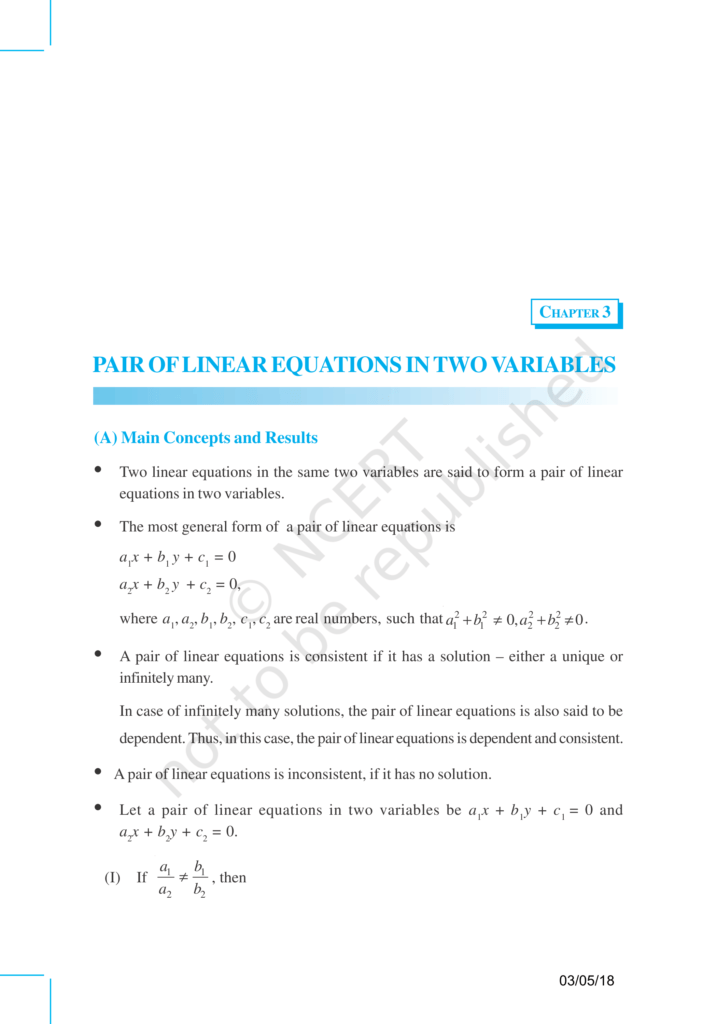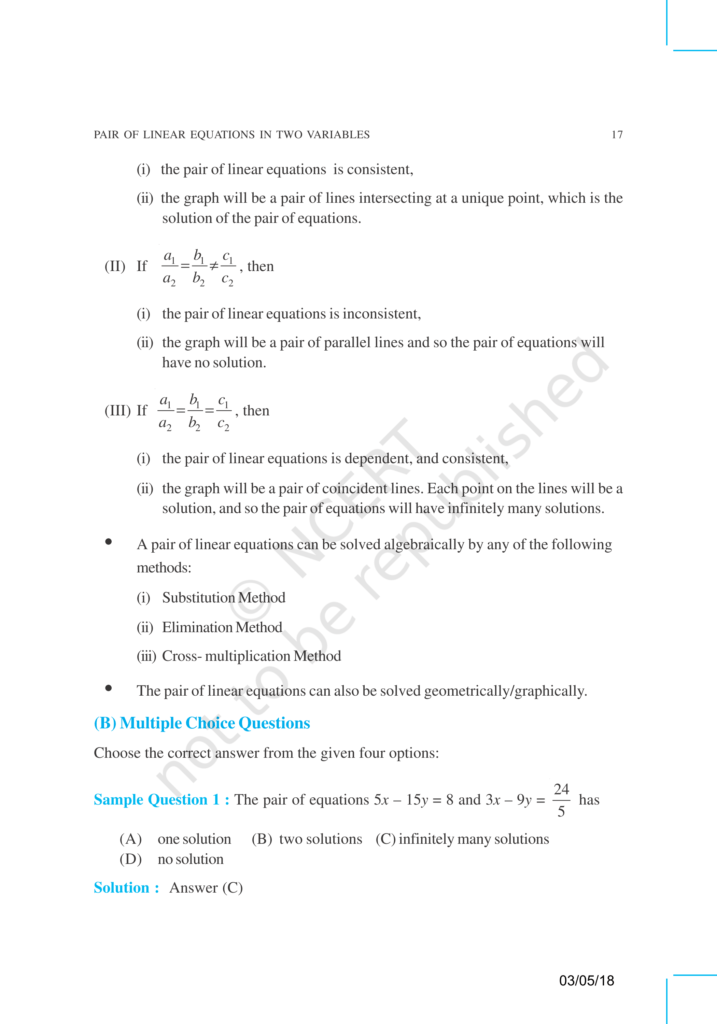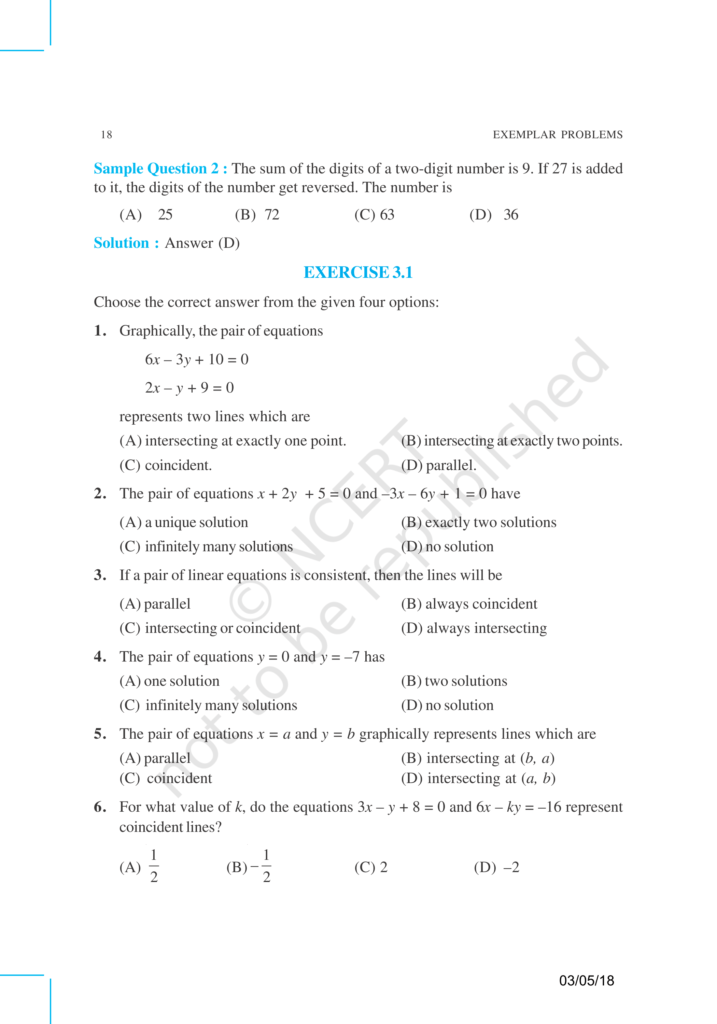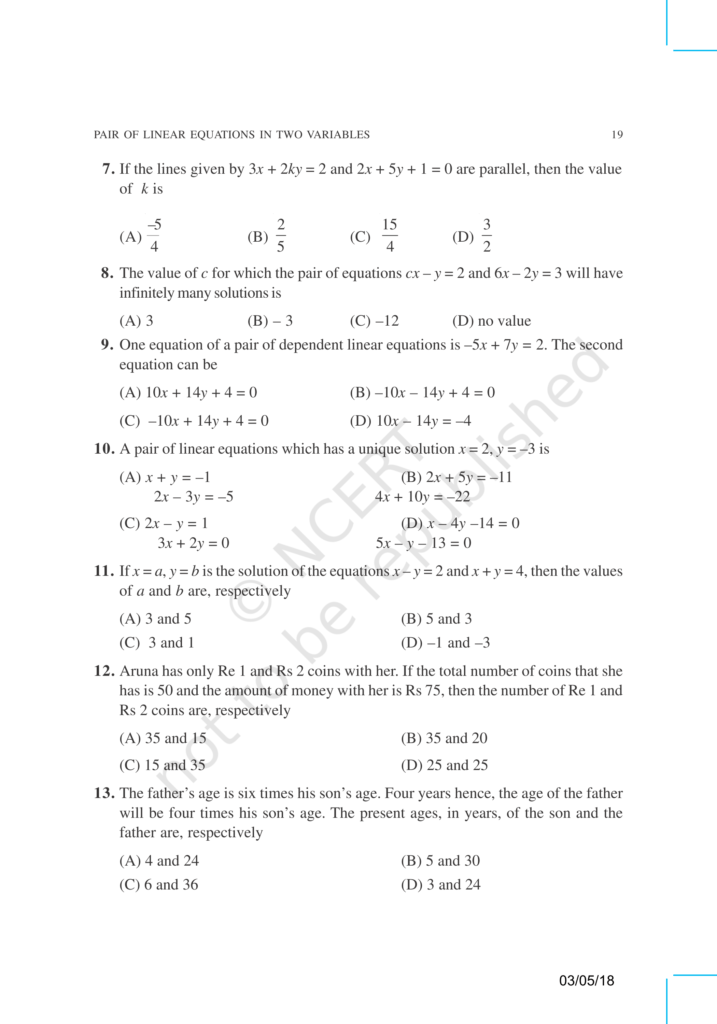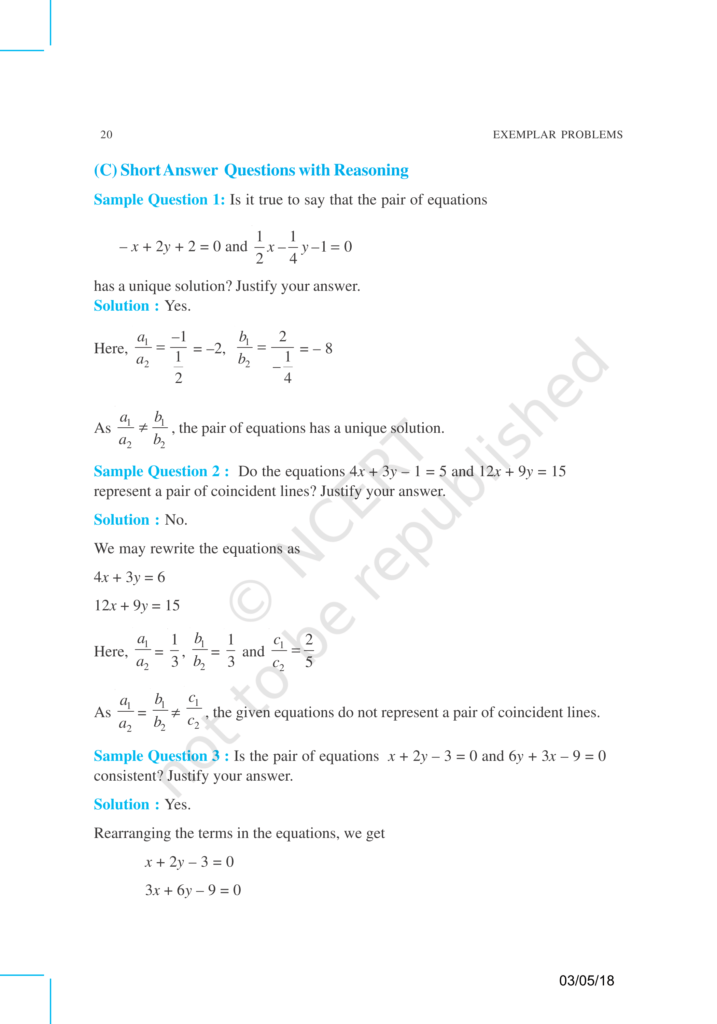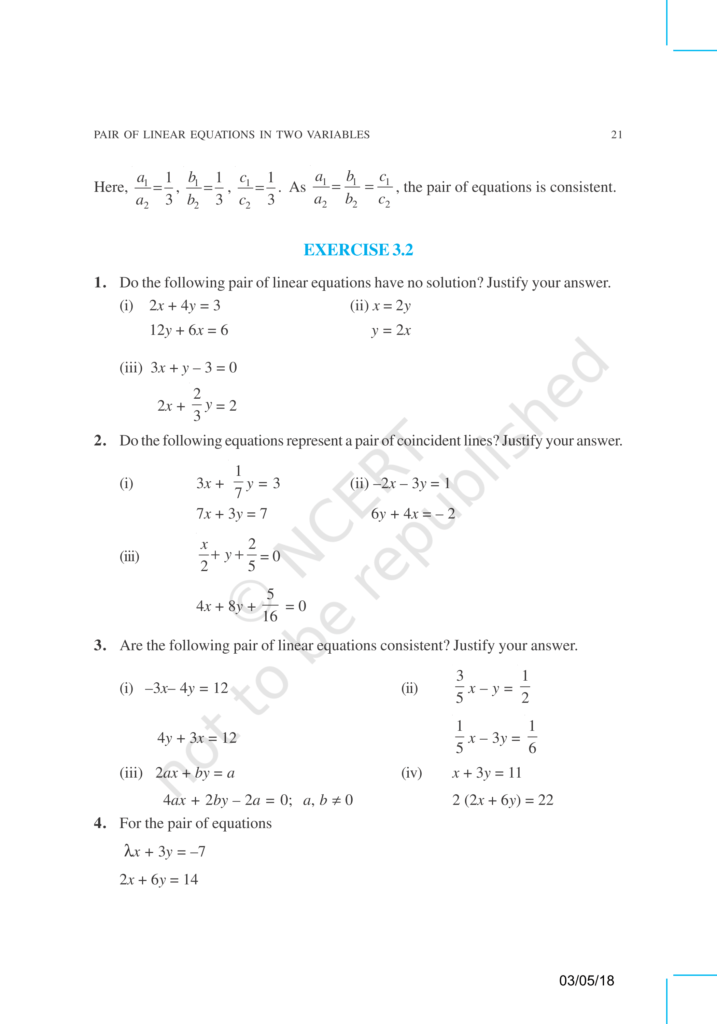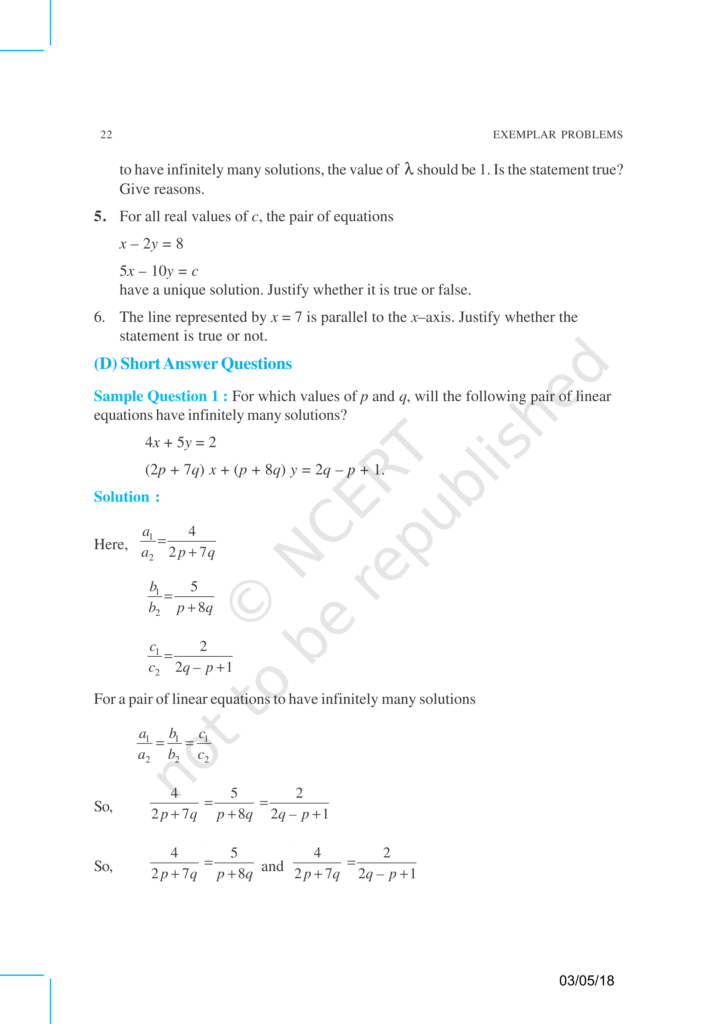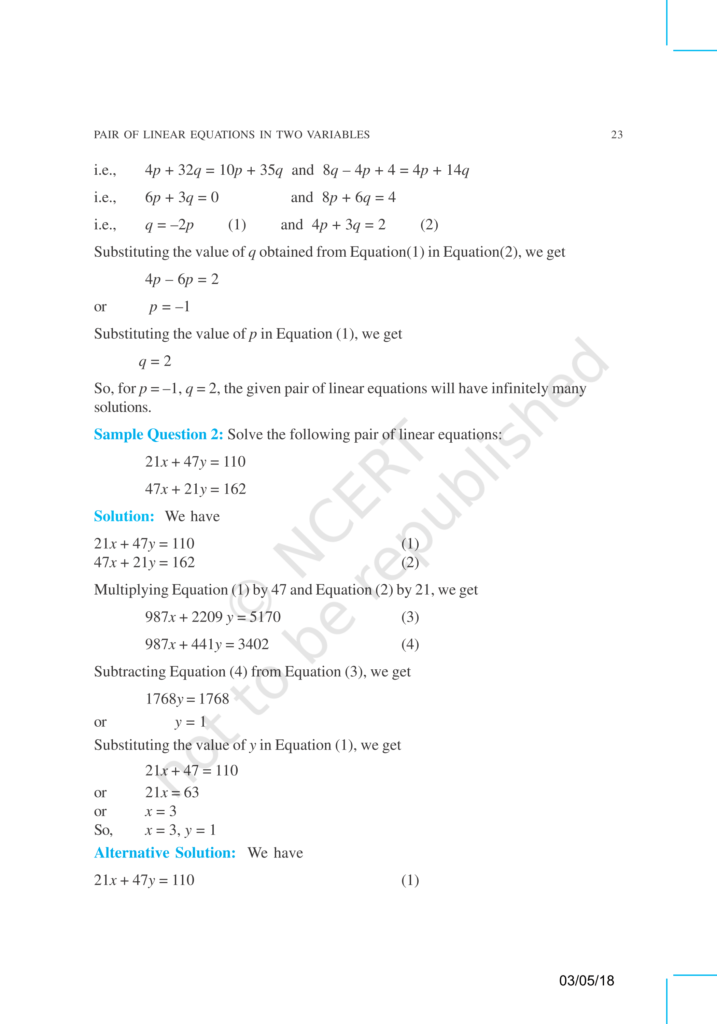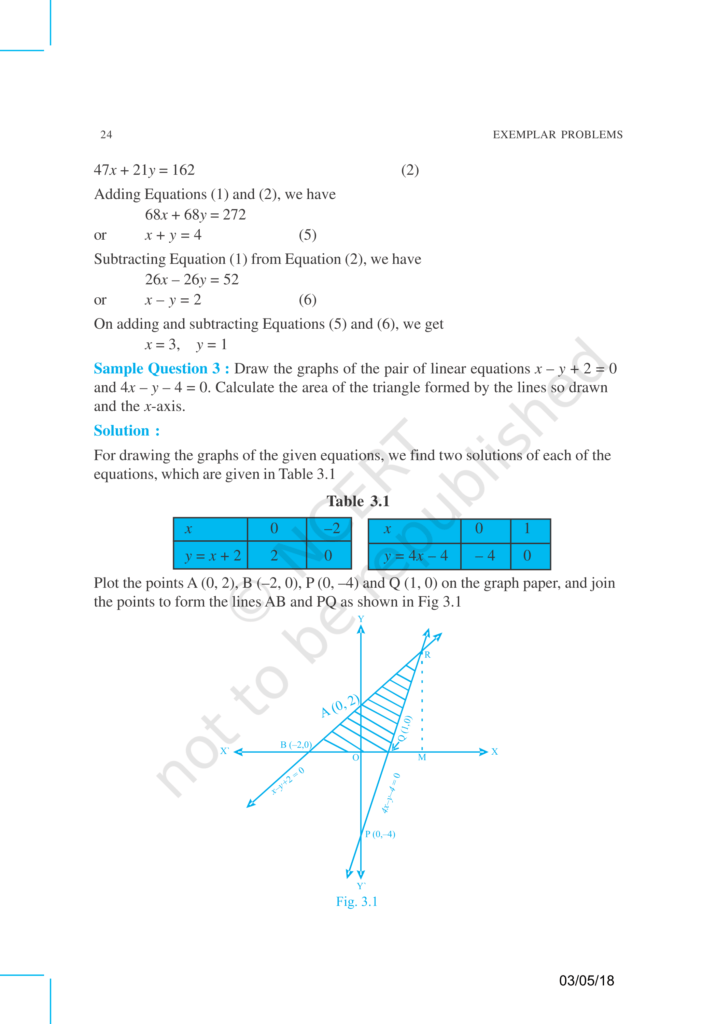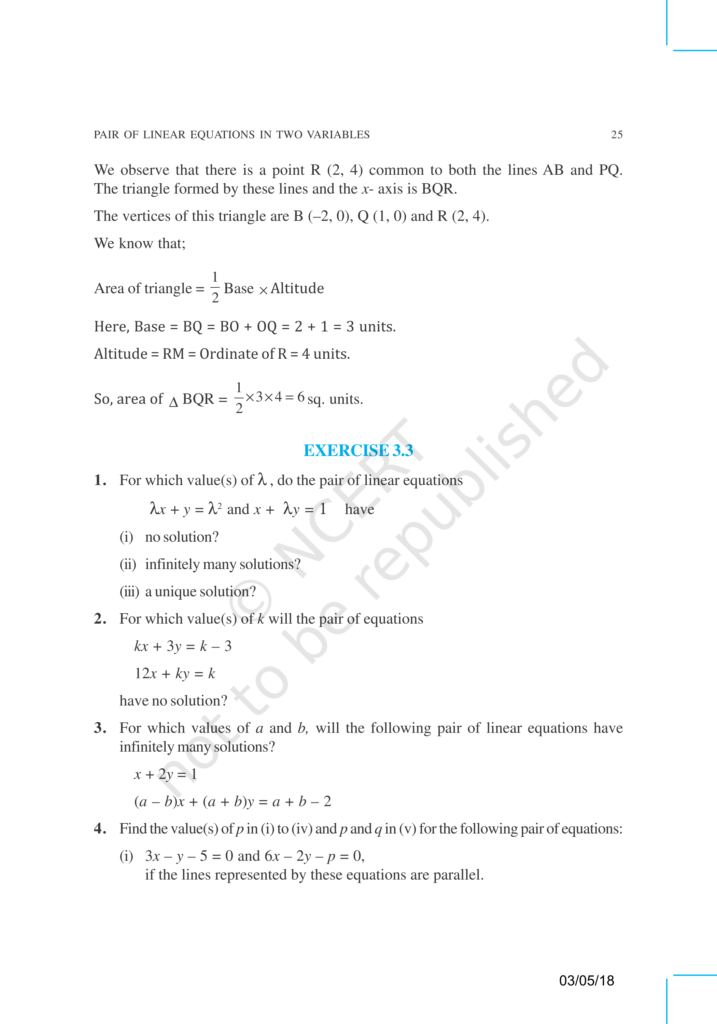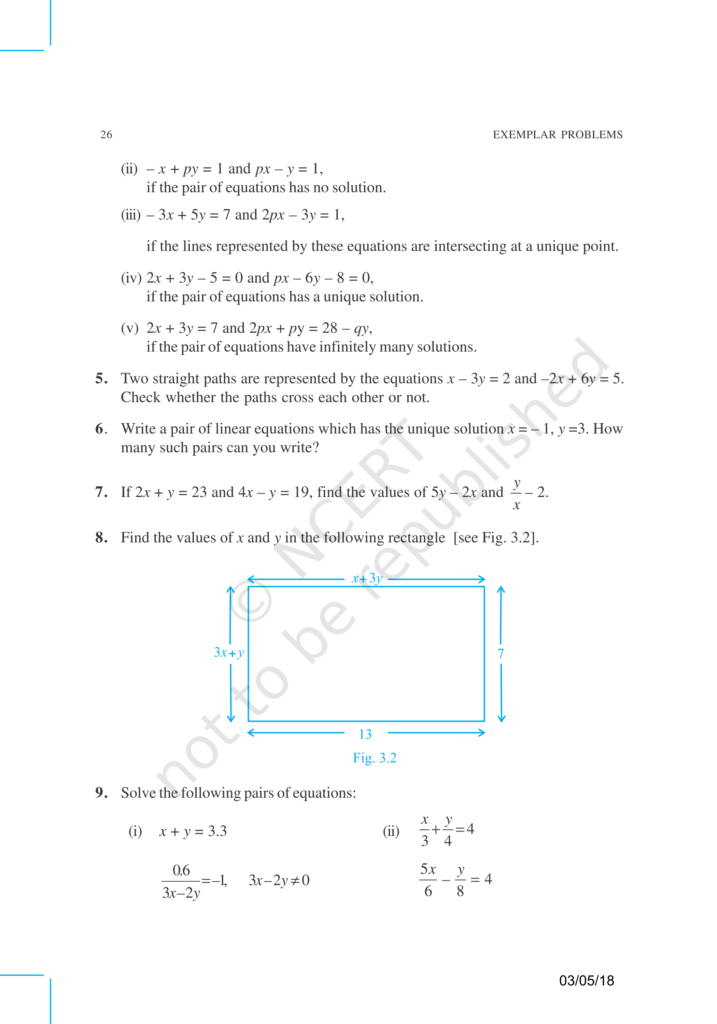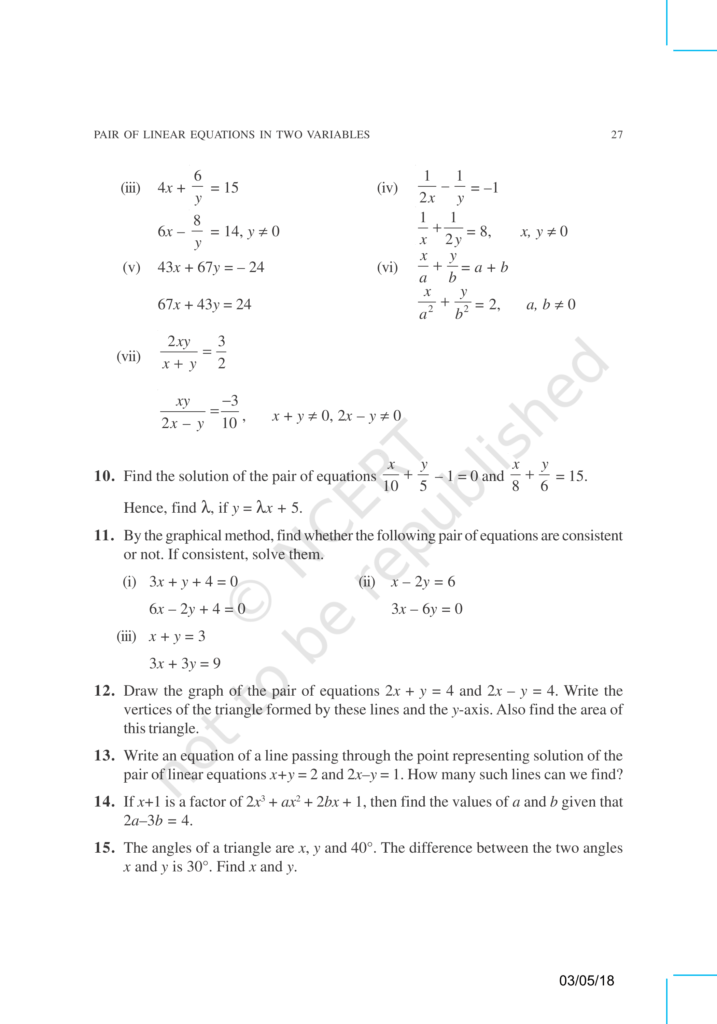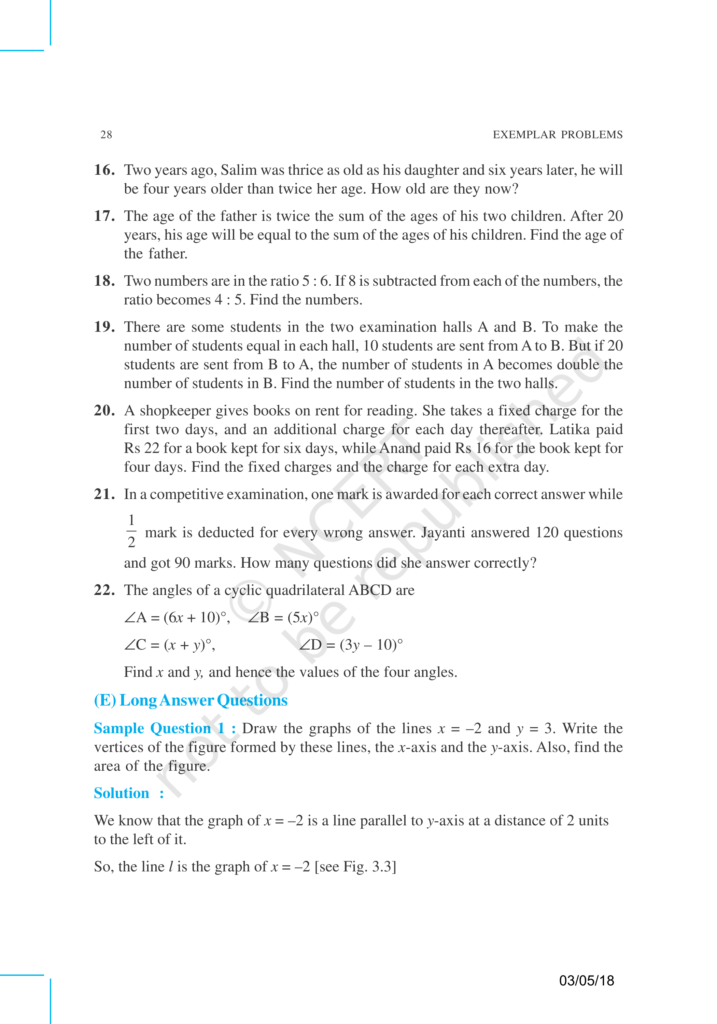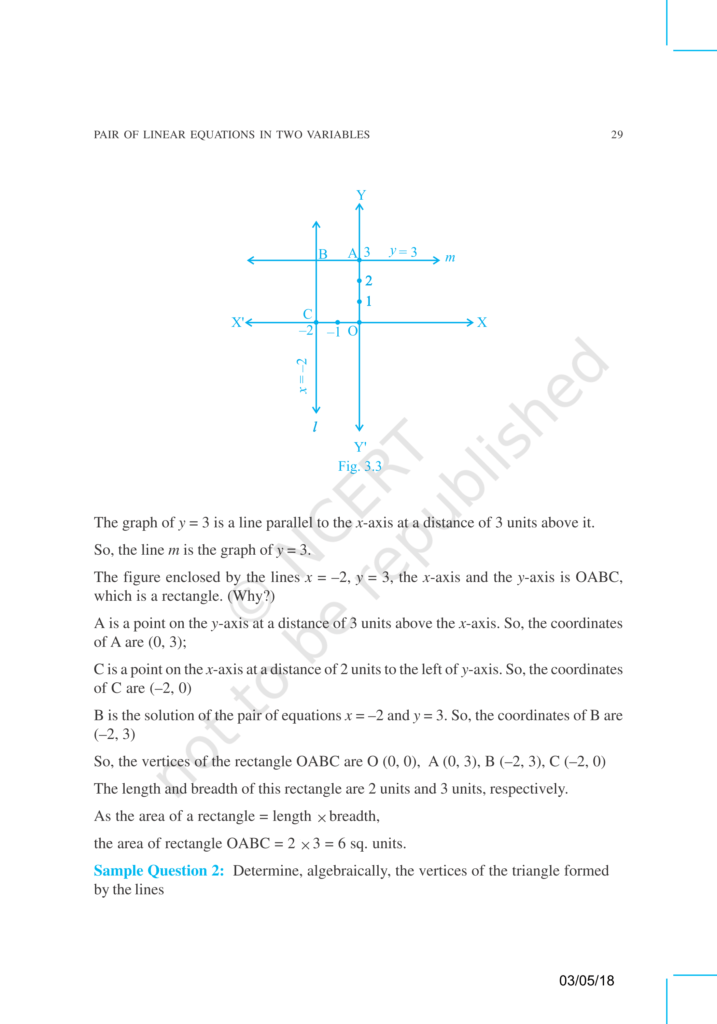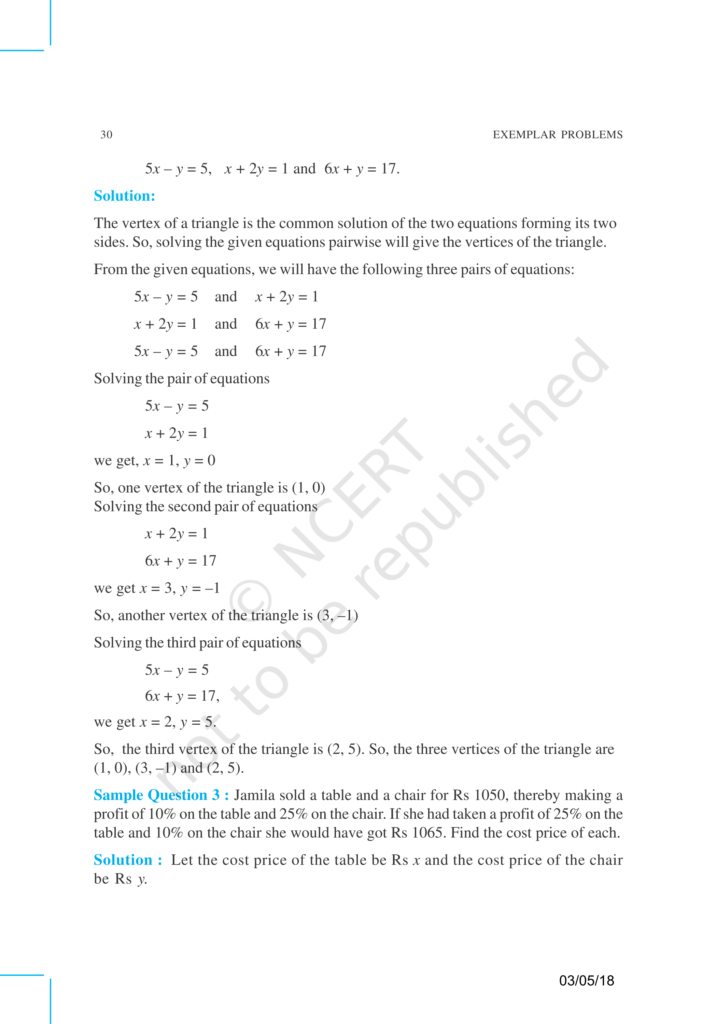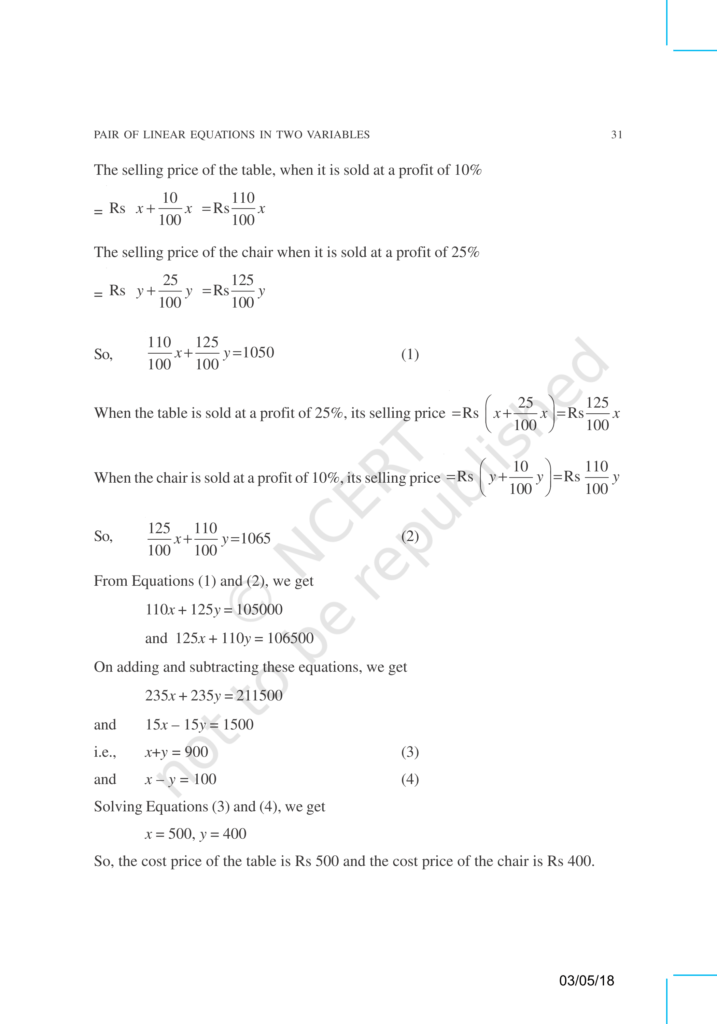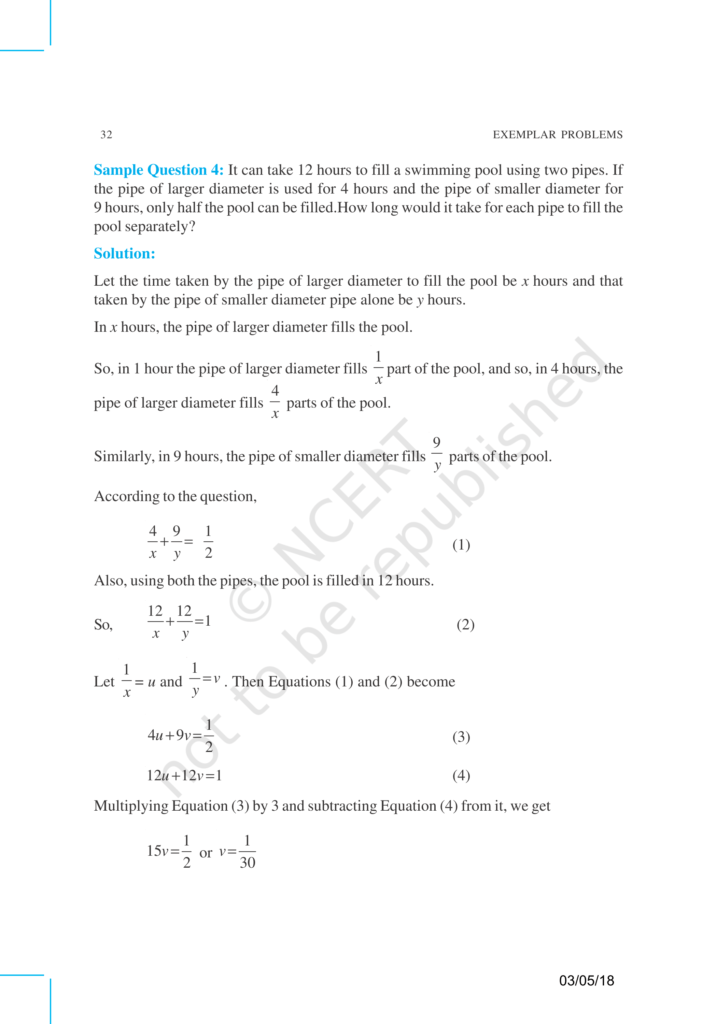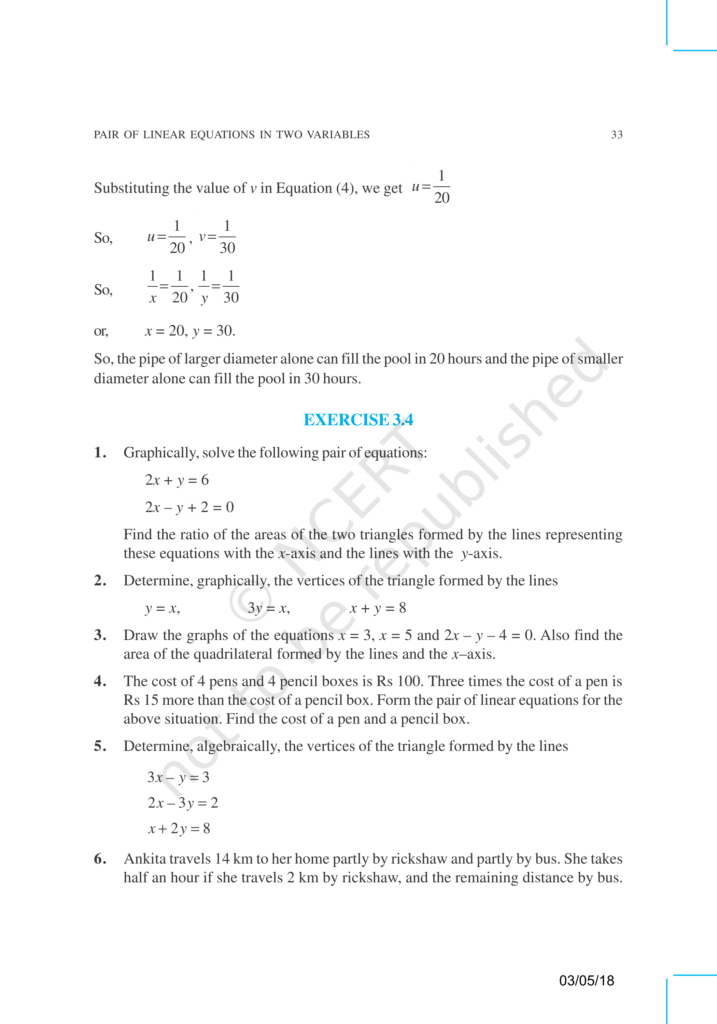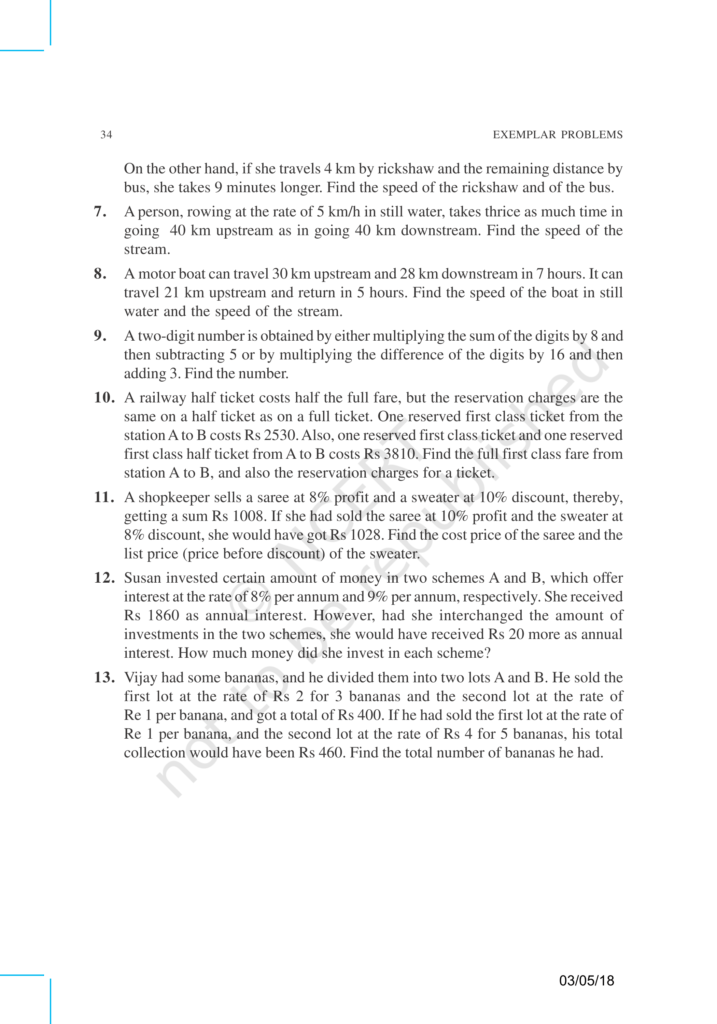# NCERT Exemplar Class 10 Maths Chapter 3 - Pair of linear Equations in Two variables - Free PDF Download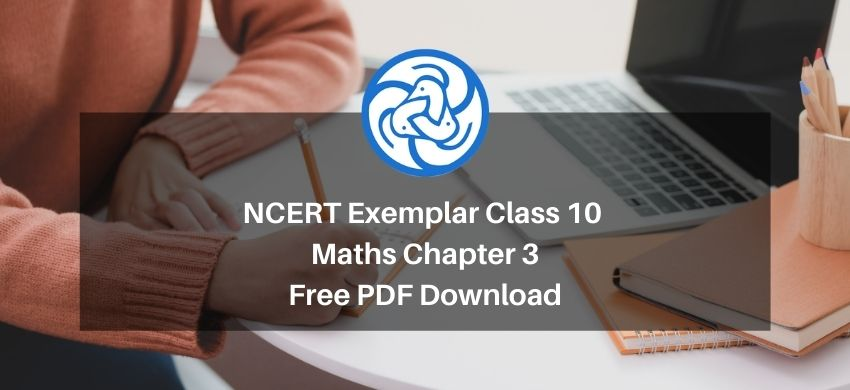Hey, are you a class 10 student? and looking for ways to download NCERT Exemplar Class 10 Maths Chapter 3 "Pair of linear Equations in Two variables"? If yes. Then read this post till the end.

If you want to score high in your Class 10 Maths exam then it is very important for you to understand and learn the topics of Class 10 Maths thoroughly.

So, without wasting more time let’s start.

### Download The PDF of NCERT Exemplars Class 10 Maths Chapter 3 "Pair of linear Equations in Two variables"